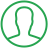# Java Random Number Between 1 And 10

Last Updated : Oct 2, 2023In this article we will show you the solution of java random number between 1 and 10, Creating random numbers is a typical operation in Java programming and is frequently necessary for different applications and simulations.

The program's behaviour is flexible and random thanks to the ability to produce random numbers inside of a defined range.

Java comes with a built-in class called "Random" that makes generating random numbers between 1 and 10 easier.

Developers can quickly produce random integers within a given range by using the Random class's methods.

The generated random numbers are guaranteed to be able to take any value between 1 and 10, both inclusive, thanks to the inclusive range of 1 to 10.

This functionality enables programmers to add randomization to their Java programs, creating a vast array of potential scenarios and results.

After that, consider the concept of a java random number between 1 and 10.

## Step By Step Guide On Java Random Number Between 1 And 10 :-

```import java.util.Random;
public class RandomNumberGenerator {
public static void main(String[] args) {
Random random = new Random();
int randomNumber = random.nextInt(10) + 1;
System.out.println("Random number between 1 and 10: " + randomNumber);
System.out.println("Generating multiple random numbers between 1 and 10:");
for (int i = 0; i < 5; i++) {
int multipleRandomNumber = random.nextInt(10) + 1;
System.out.println("Random number " + (i + 1) + ": " + multipleRandomNumber);
}
int minRange = 5;
int maxRange = 15;
int randomInRange = random.nextInt(maxRange - minRange + 1) + minRange;
System.out.println("Random number between " + minRange + " and " + maxRange + ": " + randomInRange);
}
}```
1. We import the Random class at the beginning of the code using the import java.util.Random; declaration, which enables us to produce random integers.
2. After that, the RandomNumberGenerator class is made public and has the main method, which acts as the program's entry point.
3. The line Random random = new Random(); in the main method instantiates a Random object to produce a new instance of the Random class.
4. Random numbers will be generated using this object.
5. The line with the int randomNumber = random.nextInt(10) + 1; produces a random number among 0 (inclusive) as and 10 (exclusive) with the nextInt technique of the Random class.
6. We change the range from 1 (inclusive) to 10 (inclusive) by adding 1 to the result.
7. After that, the randomNumber variable is updated with the newly produced number.
8. When a command such as System.out.println("Random number between 1 and 10: " + randomNumber); is issued, a number that is generated at random among 1 and 10 is displayed.
9. Then, several random numbers between 1 and 10 are generated and printed using a for loop.
10. The loop repeats five times, with each iteration generating a new random number using the same method.
11. The number of iterations is then recorded using i variable.
12. The required range is specified using the minRange and maxRange variables.
13. It is a random formula. A random number within the given range (inclusive) is generated by nextInt(maxRange - minRange + 1) + minRange and stored in the randomInRange variable.
14. Finally, we produce the created random number within the defined range utilising the line System.out.println("Random number between " + minRange + " and " + maxRange + ": " + randomInRange);.

## Conclusion :-

Therefore, we were able to understand the idea of a java random number between 1 and 10.

We also discovered that programmers may quickly produce random integers within a specified range by making an instance of the Random class.

The code demonstrates producing a single random number between 1 and 10, producing and publishing numerous random numbers within the same range, and producing random numbers inside a particular user-defined range.

I hope this article on java random number between 1 and 10 helps you and the steps and method mentioned above are easy to follow and implement.

##About Pragati

Experienced coding content writer who enjoys breaking down complex concepts in programming languages like Java, Python, C, and C++. Over three years of experience producing interesting and relevant content for a variety of entities. Committed to providing concise and easy-to-understand articles that assist readers in easily understanding technology and industry trends in the world of coding and software development.